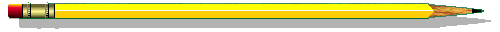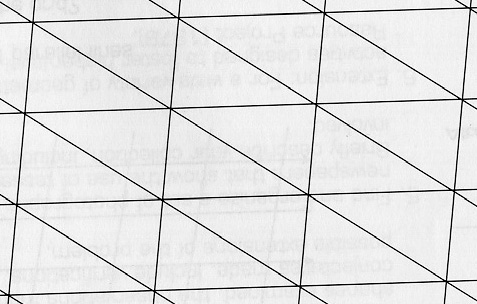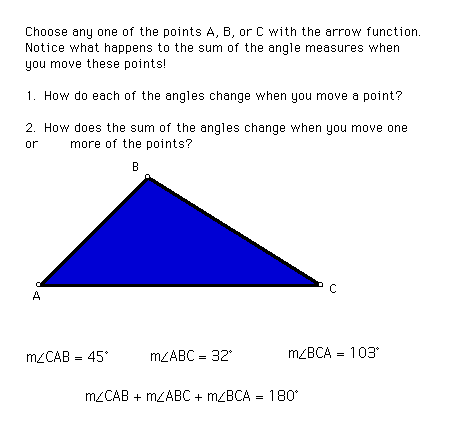The Sum of the Angles of a TriangleTriangular Grid Activity

The following grid was formed by three sets of parallel lines.You can print out the grid and complete the following activity:

• Outline one of the inner triangles on the above grid and label the angles A, B, and C.
• Choose three different color crayons, pencils, or markers.
• With the first color, color in angle A and all angles in the grid congruent to angle A.
• With the second color, color in angle B and all angles in the grid congruent to angle B.
• With the third color, color in angle C and all angles in the grid congruent to angle C.

Study the result and answer the following questions:

1) What do you observe about the angles around A, B, and C?

2) Find a straight angle. What color are the angles that make up the straight angle? Repeat this procedure for three other straight angles in the grid.

3) What colors are the angles of triangle ABC?

4) What conjecture can you make about the sum of the angles of triangle ABC?

5) Do you think this conjecture is true for all triangles? Why or why not?The Geometer's Sketchpad Activity

Explore the angles of a triangle using the Geometer's Sketchpad by clicking on the picture below.Did the conjecture you made in the triangular grid activity also hold in the Geometer's Sketchpad activity?

What can you conclude about the angles of a triangle?Conclusion